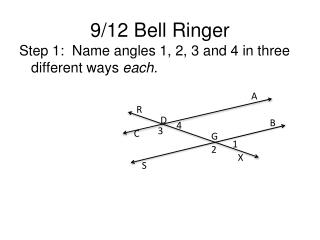DownloadDownload Presentation9/12 Bell Ringer

# 9/12 Bell Ringer

Download Presentation## 9/12 Bell Ringer

- - - - - - - - - - - - - - - - - - - - - - - - - - - E N D - - - - - - - - - - - - - - - - - - - - - - - - - - -
##### Presentation Transcript

1. 9/12 Bell Ringer A R D B 4 3 C G 1 2 X S Step 1: Name angles 1, 2, 3 and 4 in three different ways each.

2. 9/12 News and Notes • Syllabus Status • Exit Slip Grades • Announcements: Quiz 1.1 on Friday • Covering all material up to this point

3. 9/12 Agenda SWBAT identify collinear and coplanar points, parallel and perpendicular lines. • Do Now Review • Exit Slip Review • General Word Knowledge • 1 Example – Writing geometry sentences • Guided Practice – Convert the following to geometry sentences! • Independent Practice

4. New Material • Today we will talk more about point and line relationships in 2-D space. • What does it mean for two teachers to be coworkers? • Work together • Work on the same projects, do similar things.

5. New Material, cont. • Based on your understanding of what a coworker is, write down your own definition of what it means for 2 points to be collinear: • 2 points are collinear if they’re on the same line. • Now write down what you think it means for two points or lines to be coplanar: • 2 points or lines are coplanar if they’re in the same plane.

6. New Material, cont. • Now think back to the Chicago Map. Recall that it is set up like a coordinate plane. • Do Ashland and Loomis ever intersect (cross each other)? • So what are they? • PARALLEL LINES NEVER INTERSECT

7. New Material, cont. • What about Roosevelt and Loomis? How do they intersect? • They intersect at right angles • Two angles that intersect at a 90° angle are PERPENDICULAR LINES.

8. 1 Example B A • Give examples of: • Collinear points • Coplanar points • Non-collinear points • Non-coplanar points E G F K J H D C X R

9. 1 Example B A • Give examples of: • What lines are // ? EF // HJ • What lines are ? EF GK and HJ GK E G F K J H D C X R

10. Now write a sentence that details the relationship between elements B A • EF and HP • In words: EF is parallel to HP • In math notation: EF // HP F R E K P H D C O T

11. Now write a sentence that details the relationship between elements B A • EF and RK • In words: EF is perpendicular to RK • In math notation: EF RK F R E K P H D C O T

12. Now write a sentence that details the relationship between elements B A • R and H R and H are coplanar • T and K T and K are noncoplanar • H and K H and K are collinear F R E K P H D C O T • P and E • P and E are noncollinear

13. Independent Practice • You now have time now to work on the Independent practice portion of the work.

14. EXIT SLIP Answer on a sheet of loose leaf S L 1.) Give an example of perpendicular lines, using geometric notation. 2.) What is the relationship between points O and P? 3.) Describe the relationship between points K and O (hint: there are two answers for this). 4.) Which lines are parallel to each other? (Use geometric notation to describe. 5.) What type of angle is formed at a perpendicular intersection? Q J K R O N M T P